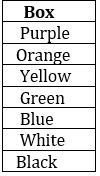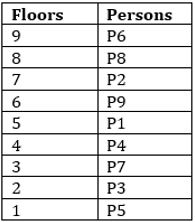Latest Banking jobs   »

# Reasoning Ability Quiz For IBPS Clerk/PO Prelims 2022- 25th August

Directions (1-5): Study the following digit-letter-symbol sequence carefully and answer the questions given below:

S ¥ U H L 2 © D \$ M # 8 C % B * V 5 & A W 4 P E + Q @ 7 F 6

Q1. How many such number are there in the above sequence, each of which is immediately preceded by a consonant and immediately followed by a symbol?
(a) None
(b) One
(c) Two
(d) Four
(e) None of these

Q2. Which of the following is 5th to the left of 17th element from the left?
(a) %
(b) C
(c) M
(d) #
(e) 8

Q3. If all the numbers are dropped in the given series, then which element will be at 10th position from right end?
(a) M
(b) V
(c) B
(d) %
(e) C

Q4. If the first fifteen elements in the above sequence are written in reverse order, then which of the following element will be 22nd from the right end?
(a) 2
(b) D
(c) P
(e) B

Q5. What should come in place of question mark in the following on the basis of above sequence?
U©L \$C# B&V ?
(a) W+P
(b) WEQ
(c) E@7
(d) W+E
(e) None of these

Directions (6-10): Read the given information carefully and answer the questions;
Seven boxes are placed one above another but not necessarily in same order. Each box painted in different colour. Only two boxes are placed between green box and purple box. Orange box is placed above green box. Only one box is placed between Blue and Black box. More than three boxes are placed between the Purple and Black box which is not placed on top. As many boxes placed between Green and White as placed between yellow and purple box. White box is not placed above yellow box.

Q6. Which of the following box is placed at top?
(a) white
(b) purple
(c) green
(d) orange
(e) None of these

Q7. Which of the following box is placed just below white box?
(a) purple
(b) yellow
(c) black
(d) green
(e) None of these

Q8. How many boxes are placed between white colour box and orange colour box?
(a) One
(b) Three
(c) Two
(d) More than three
(e) None of these

Q9. Which of the following box is placed just above purple colour box?
(a) yellow
(b) blue
(c) green
(d) white
(e)No one

Q10. Which of the following box is placed exactly between purple colour box and black colour box?
(a) yellow
(b) green
(c) purple
(d) blue
(e) black

Directions (11-15): Study the following information carefully and answer the questions given below:
Nine people viz. P1, P2, P3, P4, P5, P6, P7, P8 and P9 live in a building on nine different floors. The ground floor is numbered 1, the floor just above it is numbered 2 and so on till top floor which is numbered as 9 (but not necessarily in the same order). More than four persons are living above P4. Three persons are living between P6 and P1 who is living belowP8. P2 lives immediately above P9 and immediately below P8. P3 lives below P4 and above P5. Only one person is living between P5 and P7.

Q11. Which of the following is true regarding P1?
(a)Three persons are living below P1
(b)Only One person is living between P1 and P9
(c)P1 is living on an even numbered floor
(d)All are true
(e)Four persons are living above P1

Q12. Four of the following five are alike in a certain way and hence form a group, which of the following does not belong to the group?
(a)P6-P8
(b)P1-P9
(c)P4-P7
(d)P2-P9
(e)P3-P5

Q13. Who among the following is living on the topmost floor?
(a)P1
(b)P6
(c)P9
(d)P8
(e)None of these

Q14. How many persons are living between P4 and P5?
(a)Two
(b)Three
(c)One
(d)More than four
(e)Four

Q15. Number of persons living above P8 is same as the number of persons living below________?
(a)P4
(b)P2
(c)P1
(d)P3
(e)None of these

Solutions

S1. Ans. (c)
Sol. L 2 ©, V 5 &

S2. Ans. (e)
Sol. 5th to the left of 17th from the left= (17-5) = 12th from the left. i.e 8.

S3. Ans. (b)
S4. Ans. (d)
S5. Ans. (a)

Solutions (6-10):
Sol.S6. Ans. (b)
S7. Ans. (c)
S8. Ans. (b)
S9. Ans. (e)
S10. Ans. (b)

Solutions (11-15)
Sol.S11. Ans. (e)
S12. Ans. (b)
S13. Ans. (b)
S14. Ans. (a)
S15. Ans. (d)#### Congratulations!Download Hindu Review of October 2021: Free PDF